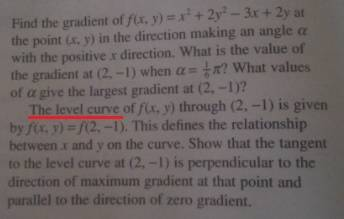# Directional derivative questionI've done the first part, but I'm stuck on the second paragraph of the question. Maybe I'm being stupid, I don't even understand exactly what is meant by, 'the level curve'.

I also don't quite understand the whole concept of directional derivative. When it says, 'the gradient in the direction making an angle A with the x-axis, how should I think of this? what does the gradient mean in this context?

because when its just a simple curve/line on an xy axis, I know what the 'gradient' means, it is literally how much 'y' changes per unit x along that curve/line. But when I'm told about a 'gradient in a direction', I'm confused.

tiny-tim
Homework Helper
hey, question dude!… what is meant by, 'the level curve'.

think of the 3D graph, z = f(x,y)

you can make a 2D contour map showing the lines of equal height

those contours are the level curvesI also don't quite understand the whole concept of directional derivative. When it says, 'the gradient in the direction making an angle A with the x-axis, how should I think of this? what does the gradient mean in this context?

the directional derivative in the direction (cosθ,sinθ) is df(kcosθ,ksinθ)/dk

it's the rate at which f increases if you go along the line y/x = tanθRay Vickson
Homework Helper
Dearly MissedI've done the first part, but I'm stuck on the second paragraph of the question. Maybe I'm being stupid, I don't even understand exactly what is meant by, 'the level curve'.

I also don't quite understand the whole concept of directional derivative. When it says, 'the gradient in the direction making an angle A with the x-axis, how should I think of this? what does the gradient mean in this context?

because when its just a simple curve/line on an xy axis, I know what the 'gradient' means, it is literally how much 'y' changes per unit x along that curve/line. But when I'm told about a 'gradient in a direction', I'm confused.

You are supposed to show your work, confused or not (those are PF rules). I don't see how you can have done the first part (which involves directional derivatives) but then claim you do not understand directional derivatives. I would need to see your work in order to grasp what is going on.

hey, question dude!think of the 3D graph, z = f(x,y)

you can make a 2D contour map showing the lines of equal height

those contours are the level curvesthe directional derivative in the direction (cosθ,sinθ) is df(kcosθ,ksinθ)/dk

it's the rate at which f increases if you go along the line y/x = tanθThanks a lot!!! I understand whats going on now. Before I was just plugging in the numbers without knowing the concepts properly.

You are supposed to show your work, confused or not (those are PF rules). I don't see how you can have done the first part (which involves directional derivatives) but then claim you do not understand directional derivatives. I would need to see your work in order to grasp what is going on.

Sorry about that, what I meant to get across, was that I understood the method in a sort of algorithmic way, but didn't understood the reason behind steps due to not understanding some basic stuff like 'level curve'. Anyway its sorted now.